# 406 Results

View
Selected filters:
• Geometry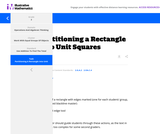Unrestricted Use
CC BY
Rating

This is a task from the Illustrative Mathematics website that is one part of a complete illustration of the standard to which it is aligned. Each task has at least one solution and some commentary that addresses important aspects of the task and its potential use.

Subject:
Geometry
Material Type:
Activity/Lab
Provider:
Illustrative Mathematics
Provider Set:
Illustrative Mathematics
Author:
Illustrative Mathematics
11/17/2020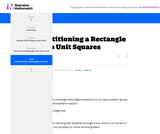Unrestricted Use
CC BY
Rating

This is a task from the Illustrative Mathematics website that is one part of a complete illustration of the standard to which it is aligned. Each task has at least one solution and some commentary that addresses important aspects of the task and its potential use.

Subject:
Geometry
Material Type:
Activity/Lab
Provider:
Illustrative Mathematics
Provider Set:
Illustrative Mathematics
Author:
Illustrative Mathematics
11/17/2020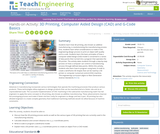Read the Fine Print
Educational Use
Rating

Students learn how 3D printing, also known as additive manufacturing, is revolutionizing the manufacturing process. First, students learn what considerations to make in the engineering design process to print an object with quality and to scale. Students learn the basic principles of how a computer-aided design (CAD) model is converted to a series of data points then turned into a program that operates the 3D printer. The activity takes students through a step-by-step process on how a computer can control a manufacturing process through defined data points. Within this activity, students also learn how to program using basic G-code to create a wireframe 3D shapes that can be read by a 3D printer or computer numerical control (CNC) machine.

Subject:
Computer Science
Geometry
Measurement and Data
Engineering
Material Type:
Activity/Lab
Provider:
TeachEngineering
Provider Set:
Activities
Author:
Matthew Jourden
05/04/2019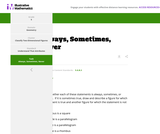Unrestricted Use
CC BY
Rating

This is a task from the Illustrative Mathematics website that is one part of a complete illustration of the standard to which it is aligned. Each task has at least one solution and some commentary that addresses important aspects of the task and its potential use.

Subject:
Geometry
Material Type:
Activity/Lab
Provider:
Illustrative Mathematics
Provider Set:
Illustrative Mathematics
Author:
Illustrative Mathematics
11/17/2020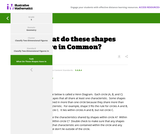Unrestricted Use
CC BY
Rating

This is a task from the Illustrative Mathematics website that is one part of a complete illustration of the standard to which it is aligned. Each task has at least one solution and some commentary that addresses important aspects of the task and its potential use.

Subject:
Geometry
Material Type:
Activity/Lab
Provider:
Illustrative Mathematics
Provider Set:
Illustrative Mathematics
Author:
Illustrative Mathematics
11/17/2020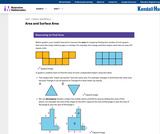Unrestricted Use
CC BY
Rating

Family facing 6th Grade math unit focusing on area and surface area.

Subject:
Geometry
Material Type:
Unit of Study
Provider:
Illustrative Mathematics
11/17/2020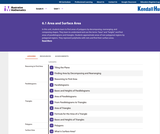Unrestricted Use
CC BY
Rating

In this unit, students learn to find areas of polygons by decomposing, rearranging, and composing shapes. They learn to understand and use the terms “base” and “height,” and find areas of parallelograms and triangles. Students approximate areas of non-polygonal regions by polygonal regions. They represent polyhedra with nets and find their surface areas.

Subject:
Geometry
Material Type:
Unit of Study
Provider:
Illustrative Mathematics
11/17/2020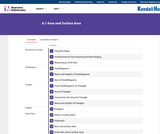Unrestricted Use
CC BY
Rating

Student facing 6th Grade math unit focusing on area and surface area.

Subject:
Geometry
Material Type:
Unit of Study
Provider:
Illustrative Mathematics
11/17/2020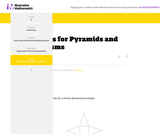Unrestricted Use
CC BY
Rating

This is a task from the Illustrative Mathematics website that is one part of a complete illustration of the standard to which it is aligned. Each task has at least one solution and some commentary that addresses important aspects of the task and its potential use.

Subject:
Geometry
Material Type:
Activity/Lab
Provider:
Illustrative Mathematics
Provider Set:
Illustrative Mathematics
Author:
Illustrative Mathematics
11/17/2020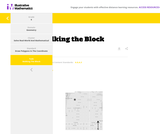Unrestricted Use
CC BY
Rating

This is a task from the Illustrative Mathematics website that is one part of a complete illustration of the standard to which it is aligned. Each task has at least one solution and some commentary that addresses important aspects of the task and its potential use.

Subject:
Geometry
Material Type:
Activity/Lab
Provider:
Illustrative Mathematics
Provider Set:
Illustrative Mathematics
Author:
Illustrative Mathematics
11/17/2020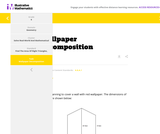Unrestricted Use
CC BY
Rating

This is a task from the Illustrative Mathematics website that is one part of a complete illustration of the standard to which it is aligned. Each task has at least one solution and some commentary that addresses important aspects of the task and its potential use.

Subject:
Geometry
Material Type:
Activity/Lab
Provider:
Illustrative Mathematics
Provider Set:
Illustrative Mathematics
Author:
Illustrative Mathematics
11/17/2020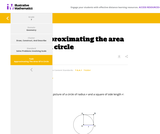Unrestricted Use
CC BY
Rating

This is a task from the Illustrative Mathematics website that is one part of a complete illustration of the standard to which it is aligned. Each task has at least one solution and some commentary that addresses important aspects of the task and its potential use.

Subject:
Geometry
Material Type:
Activity/Lab
Provider:
Illustrative Mathematics
Provider Set:
Illustrative Mathematics
Author:
Illustrative Mathematics
11/17/2020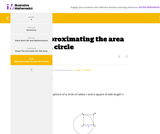Unrestricted Use
CC BY
Rating

This is a task from the Illustrative Mathematics website that is one part of a complete illustration of the standard to which it is aligned. Each task has at least one solution and some commentary that addresses important aspects of the task and its potential use.

Subject:
Geometry
Material Type:
Activity/Lab
Provider:
Illustrative Mathematics
Provider Set:
Illustrative Mathematics
Author:
Illustrative Mathematics
11/17/2020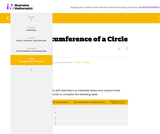Unrestricted Use
CC BY
Rating

This is a task from the Illustrative Mathematics website that is one part of a complete illustration of the standard to which it is aligned. Each task has at least one solution and some commentary that addresses important aspects of the task and its potential use.

Subject:
Geometry
Material Type:
Activity/Lab
Provider:
Illustrative Mathematics
Provider Set:
Illustrative Mathematics
Author:
Illustrative Mathematics
11/17/2020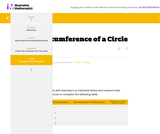Unrestricted Use
CC BY
Rating

This is a task from the Illustrative Mathematics website that is one part of a complete illustration of the standard to which it is aligned. Each task has at least one solution and some commentary that addresses important aspects of the task and its potential use.

Subject:
Geometry
Material Type:
Activity/Lab
Provider:
Illustrative Mathematics
Provider Set:
Illustrative Mathematics
Author:
Illustrative Mathematics
11/17/2020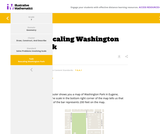Unrestricted Use
CC BY
Rating

This is a task from the Illustrative Mathematics website that is one part of a complete illustration of the standard to which it is aligned. Each task has at least one solution and some commentary that addresses important aspects of the task and its potential use.

Subject:
Geometry
Material Type:
Activity/Lab
Provider:
Illustrative Mathematics
Provider Set:
Illustrative Mathematics
Author:
Illustrative Mathematics
11/17/2020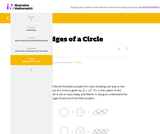Unrestricted Use
CC BY
Rating

This is a task from the Illustrative Mathematics website that is one part of a complete illustration of the standard to which it is aligned. Each task has at least one solution and some commentary that addresses important aspects of the task and its potential use.

Subject:
Geometry
Material Type:
Activity/Lab
Provider:
Illustrative Mathematics
Provider Set:
Illustrative Mathematics
Author:
Illustrative Mathematics
11/17/2020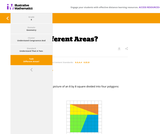Unrestricted Use
CC BY
Rating

This is a task from the Illustrative Mathematics website that is one part of a complete illustration of the standard to which it is aligned. Each task has at least one solution and some commentary that addresses important aspects of the task and its potential use.

Subject:
Geometry
Material Type:
Activity/Lab
Provider:
Illustrative Mathematics
Provider Set:
Illustrative Mathematics
Author:
Illustrative Mathematics
11/17/2020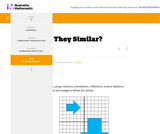Unrestricted Use
CC BY
Rating

This is a task from the Illustrative Mathematics website that is one part of a complete illustration of the standard to which it is aligned. Each task has at least one solution and some commentary that addresses important aspects of the task and its potential use.

Subject:
Geometry
Material Type:
Activity/Lab
Provider:
Illustrative Mathematics
Provider Set:
Illustrative Mathematics
Author:
Illustrative Mathematics
11/17/2020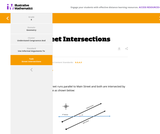Unrestricted Use
CC BY
Rating

This is a task from the Illustrative Mathematics website that is one part of a complete illustration of the standard to which it is aligned. Each task has at least one solution and some commentary that addresses important aspects of the task and its potential use.

Subject:
Geometry
Material Type:
Activity/Lab
Provider:
Illustrative Mathematics
Provider Set:
Illustrative Mathematics
Author:
Illustrative Mathematics
11/17/2020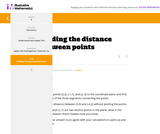Unrestricted Use
CC BY
Rating

This is a task from the Illustrative Mathematics website that is one part of a complete illustration of the standard to which it is aligned. Each task has at least one solution and some commentary that addresses important aspects of the task and its potential use.

Subject:
Geometry
Material Type:
Activity/Lab
Provider:
Illustrative Mathematics
Provider Set:
Illustrative Mathematics
Author:
Illustrative Mathematics
11/17/2020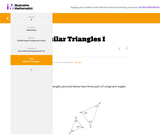Unrestricted Use
CC BY
Rating

This is a task from the Illustrative Mathematics website that is one part of a complete illustration of the standard to which it is aligned. Each task has at least one solution and some commentary that addresses important aspects of the task and its potential use.

Subject:
Geometry
Material Type:
Activity/Lab
Provider:
Illustrative Mathematics
Provider Set:
Illustrative Mathematics
Author:
Illustrative Mathematics
11/17/2020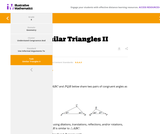Unrestricted Use
CC BY
Rating

This is a task from the Illustrative Mathematics website that is one part of a complete illustration of the standard to which it is aligned. Each task has at least one solution and some commentary that addresses important aspects of the task and its potential use.

Subject:
Geometry
Material Type:
Activity/Lab
Provider:
Illustrative Mathematics
Provider Set:
Illustrative Mathematics
Author:
Illustrative Mathematics
11/17/2020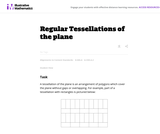Unrestricted Use
CC BY
Rating

This task examines the ways in which the plane can be covered by regular polygons in a very strict arrangement called a regular tessellation. These tessellations are studied here using algebra, which enters the picture via the formula for the measure of the interior angles of a regular polygon (which should therefore be introduced or reviewed before beginning the task). The goal of the task is to use algebra in order to understand which tessellations of the plane with regular polygons are possible.

Subject:
Mathematics
Algebra
Geometry
Material Type:
Activity/Lab
Provider:
Illustrative Mathematics
Provider Set:
Illustrative Mathematics
Author:
Illustrative Mathematics
01/21/2013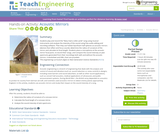Read the Fine Print
Educational Use
Rating

Students play and record the “Mary Had a Little Lamb” song using musical instruments and analyze the intensity of the sound using free audio editing and recording software. Then they use hollow Styrofoam half-spheres as acoustic mirrors (devices that reflect and focus sound), determine the radius of curvature of the mirror and calculate its focal length. Students place a microphone at the acoustic mirror focal point, re-record their songs, and compare the sound intensity on plot spectrums generated from their recordings both with and without the acoustic mirrors. A worksheet and KWL chart are provided.

Subject:
Mathematics
Geometry
Physical Science
Physics
Material Type:
Activity/Lab
Provider:
TeachEngineering
Author:
Nick Breen
Steven C. Thedford
02/17/2021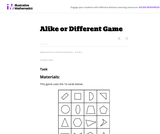Unrestricted Use
CC BY
Rating

In this game activity students practice comparing shapes and naming something that is alike or different about them.

Subject:
Mathematics
Geometry
Material Type:
Activity/Lab
Provider:
Illustrative Mathematics
Provider Set:
Illustrative Mathematics
Author:
Illustrative Mathematics
05/01/2012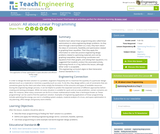Read the Fine Print
Educational Use
Rating

Students learn about linear programming (also called linear optimization) to solve engineering design problems. As they work through a word problem as a class, they learn about the ideas of constraints, feasibility and optimization related to graphing linear equalities. Then they apply this information to solve two practice engineering design problems related to optimizing materials and cost by graphing inequalities, determining coordinates and equations from their graphs, and solving their equations. It is suggested that students conduct the associated activity, Optimizing Pencils in a Tray, before this lesson, although either order is acceptable.

Subject:
Mathematics
Algebra
Geometry
Material Type:
Lesson
Provider:
TeachEngineering
Provider Set:
TeachEngineering
Author:
Andi Vicksman
Malinda Zarske
Nathan Coyle
Russell Anderson
Ryan Sullivan
02/17/2021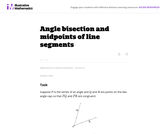Unrestricted Use
CC BY
Rating

This task provides a construction of the angle bisector of an angle by reducing it to the bisection of an angle to finding the midpoint of a line segment. It is worth observing the symmetry -- for both finding midpoints and bisecting angles, the goal is to cut an object into two equal parts. The conclusion of this task is that they are, in a sense, of exactly equivalent difficulty -- bisecting a segment allows us to bisect and angle (part a) and, conversely, bisecting an angle allows us to bisect a segment (part b). In addition to seeing how these two constructions are related, the task also provides an opportunity for students to use two different triangle congruence criteria: SSS and SAS.

Subject:
Mathematics
Geometry
Material Type:
Activity/Lab
Provider:
Illustrative Mathematics
Provider Set:
Illustrative Mathematics
Author:
Illustrative Mathematics
01/11/2013Conditional Remix & Share Permitted
CC BY-NC-SA
Rating

This short video and interactive assessment activity is designed to give fourth graders an overview of classification of angles.

Subject:
Mathematics
Geometry
Material Type:
Assessment
Interactive
Lecture
Provider:
CK-12 Foundation
Provider Set:
CK-12 Elementary Math
11/18/2020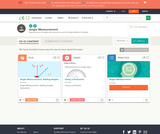Conditional Remix & Share Permitted
CC BY-NC-SA
Rating

This short video and interactive assessment activity is designed to give fourth graders an overview of measurement of angles.

Subject:
Mathematics
Geometry
Material Type:
Assessment
Interactive
Lecture
Provider:
CK-12 Foundation
Provider Set:
CK-12 Elementary Math
11/18/2020Read the Fine Print
Educational Use
Rating

Find out how angles and symmetry come into play in the game of pool in this video adapted from Annenberg Learner&rsquo;s Learning Math: Measurement.

Subject:
Mathematics
Geometry
Chemistry
Life Science
Material Type:
Lesson
Provider:
PBS LearningMedia
Author:
U.S. Department of Education
WGBH Educational Foundation
06/18/2012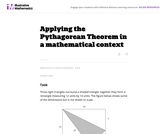Unrestricted Use
CC BY
Rating

This task requires students to apply the Pythagorean Theorem.

Subject:
Mathematics
Geometry
Material Type:
Activity/Lab
Provider:
Illustrative Mathematics
Provider Set:
Illustrative Mathematics
Author:
Illustrative Mathematics
05/01/2012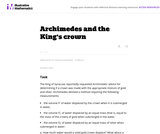Unrestricted Use
CC BY
Rating

The famous story of Archimedes running through the streets of Syracuse (in Sicily during the third century bc) shouting ''Eureka!!!'' (I have found it) reportedly occurred after he solved this problem. The problem combines the ideas of ratio and proportion within the context of density of matter.

Subject:
Mathematics
Geometry
Material Type:
Activity/Lab
Provider:
Illustrative Mathematics
Provider Set:
Illustrative Mathematics
Author:
Illustrative Mathematics
01/04/2013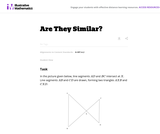Unrestricted Use
CC BY
Rating

In this problem, students are given a picture of two triangles that appear to be similar, but whose similarity cannot be proven without further information. Asking students to provide a sequence of similarity transformations that maps one triangle to the other focuses them on the work of standard G-SRT.2, using the definition of similarity in terms of similarity transformations.

Subject:
Mathematics
Geometry
Trigonometry
Material Type:
Activity/Lab
Provider:
Illustrative Mathematics
Provider Set:
Illustrative Mathematics
Author:
Illustrative Mathematics
05/01/2012Conditional Remix & Share Permitted
CC BY-NC-SA
Rating

This short video and interactive assessment activity is designed to give fourth graders an overview of composite figures composed of squares and rectangles.

Subject:
Mathematics
Geometry
Material Type:
Assessment
Interactive
Lecture
Provider:
CK-12 Foundation
Provider Set:
CK-12 Elementary Math
11/18/2020Conditional Remix & Share Permitted
CC BY-NC-SA
Rating

This short video and interactive assessment activity is designed to give fourth graders an overview of area and perimeter of rectangle.

Subject:
Mathematics
Geometry
Material Type:
Assessment
Interactive
Lecture
Provider:
CK-12 Foundation
Provider Set:
CK-12 Elementary Math
11/18/2020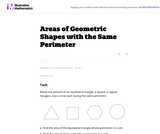Unrestricted Use
CC BY
Rating

This problem is part of a very rich tradition of problems looking to maximize the area enclosed by a shape with fixed perimeter. Only three shapes are considered here because the problem is difficult for more irregular shapes.

Subject:
Mathematics
Geometry
Material Type:
Activity/Lab
Provider:
Illustrative Mathematics
Provider Set:
Illustrative Mathematics
Author:
Illustrative Mathematics
01/20/2013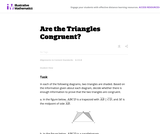Unrestricted Use
CC BY
Rating

The purpose of this task is primarily assessment-oriented, asking students to demonstrate knowledge of how to determine the congruency of triangles.

Subject:
Mathematics
Geometry
Material Type:
Activity/Lab
Provider:
Illustrative Mathematics
Provider Set:
Illustrative Mathematics
Author:
Illustrative Mathematics
05/01/2012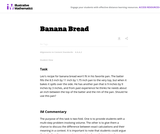Unrestricted Use
CC BY
Rating

The purpose of this task is to provide students with a multi-step problem involving volume and to give them a chance to discuss the difference between exact calculations and their meaning in a context.

Subject:
Mathematics
Geometry
Material Type:
Activity/Lab
Provider:
Illustrative Mathematics
Provider Set:
Illustrative Mathematics
Author:
Illustrative Mathematics
05/01/2012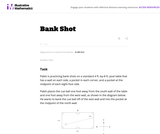Unrestricted Use
CC BY
Rating

This task asks students to use similarity to solve a problem in a context that will be familiar to many, though most students are accustomed to using intuition rather than geometric reasoning to set up the shot.

Subject:
Mathematics
Geometry
Trigonometry
Material Type:
Activity/Lab
Provider:
Illustrative Mathematics
Provider Set:
Illustrative Mathematics
Author:
Illustrative Mathematics
08/21/2012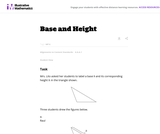Unrestricted Use
CC BY
Rating

The purpose of this task is to help students understand what is meant by a base and its corresponding height in a triangle and to be able to correctly identify all three base-height pairs.

Subject:
Mathematics
Geometry
Material Type:
Activity/Lab
Provider:
Illustrative Mathematics
Provider Set:
Illustrative Mathematics
Author:
Illustrative Mathematics
05/01/2012Conditional Remix & Share Permitted
CC BY-NC-SA
Rating

This short video and interactive assessment activity is designed to teach second graders an overview of lines, curves, vertices, and sides of 2d shapes.

Subject:
Mathematics
Geometry
Material Type:
Assessment
Interactive
Lecture
Provider:
CK-12 Foundation
Provider Set:
CK-12 Elementary Math
11/18/2020Conditional Remix & Share Permitted
CC BY-NC-SA
Rating

This short video and interactive assessment activity is designed to teach third graders an overview of lines, curves, vertices, and sides of 2d shapes.

Subject:
Mathematics
Geometry
Material Type:
Assessment
Interactive
Lecture
Provider:
CK-12 Foundation
Provider Set:
CK-12 Elementary Math
11/18/2020Conditional Remix & Share Permitted
CC BY-NC-SA
Rating

This short video and interactive assessment activity is designed to give fourth graders an overview of simple figure patterns.

Subject:
Mathematics
Geometry
Material Type:
Assessment
Interactive
Lecture
Provider:
CK-12 Foundation
Provider Set:
CK-12 Elementary Math
11/18/2020Conditional Remix & Share Permitted
CC BY-NC-SA
Rating

This short video and interactive assessment activity is designed to teach third graders an overview of simple figure patterns.

Subject:
Mathematics
Geometry
Material Type:
Assessment
Interactive
Lecture
Provider:
CK-12 Foundation
Provider Set:
CK-12 Elementary Math
11/18/2020Conditional Remix & Share Permitted
CC BY-NC-SA
Rating

This short video and interactive assessment activity is designed to give fifth graders an overview of simple figure patterns.

Subject:
Mathematics
Geometry
Material Type:
Assessment
Interactive
Lecture
Provider:
CK-12 Foundation
Provider Set:
CK-12 Elementary Math
11/18/2020Conditional Remix & Share Permitted
CC BY-NC-SA
Rating

This short video and interactive assessment activity is designed to teach second graders an overview of simple figure patterns.

Subject:
Mathematics
Geometry
Material Type:
Assessment
Interactive
Lecture
Provider:
CK-12 Foundation
Provider Set:
CK-12 Elementary Math
11/18/2020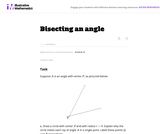Unrestricted Use
CC BY
Rating

This task provides the most famous construction to bisect a given angle. It applies when the angle is not 180 degrees.

Subject:
Mathematics
Geometry
Material Type:
Activity/Lab
Provider:
Illustrative Mathematics
Provider Set:
Illustrative Mathematics
Author:
Illustrative Mathematics
01/11/2013
Remix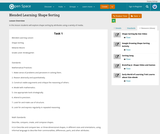Unrestricted Use
CC BY
Rating

In this lesson students will explore shape sorting by attributes using a variety of media.

Subject:
Mathematics
Geometry
Material Type:
Lesson Plan
Author:
Melanie Moore
08/13/2019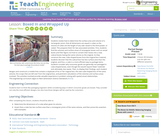Read the Fine Print
Educational Use
Rating

Students find the volume and surface area of a rectangular box (e.g., a cereal box), and then figure out how to convert that box into a new, cubical box having the same volume as the original. As they construct the new, cube-shaped box from the original box material, students discover that the cubical box has less surface area than the original, and thus, a cube is a more efficient way to package things. Students then consider why consumer goods generally aren't packaged in cube-shaped boxes, even though they would require less material to produce and ultimately, less waste to discard. To display their findings, each student designs and constructs a mobile that contains a duplicate of his or her original box, the new cube-shaped box of the same volume, the scraps that are left over from the original box, and pertinent calculations of the volumes and surface areas involved. The activities involved provide valuable experience in problem solving with spatial-visual relationships.

Subject:
Geometry
Engineering
Material Type:
Activity/Lab
Lesson Plan
Provider:
TeachEngineering
Provider Set:
TeachEngineering
Author:
Mary R. Hebrank
09/18/2014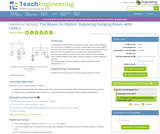Read the Fine Print
Educational Use
Rating

To display the results from the previous activity, each student designs and constructs a mobile that contains a duplicate of his or her original box, the new cube-shaped box of the same volume, the scraps that are left over from the original box, and pertinent calculations of the volumes and surface areas involved. They problem solve and apply their understanding of see-saws and lever systems to create balanced mobiles.

Subject:
Geometry
Engineering
Material Type:
Activity/Lab
Provider:
TeachEngineering
Provider Set:
TeachEngineering
Author:
Mary R. Hebrank
10/14/2015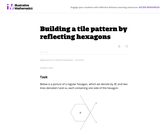Unrestricted Use
CC BY
Rating

This task applies reflections to a regular hexagon to construct a pattern of six hexagons enclosing a seventh: the focus of the task is on using the properties of reflections to deduce this seven hexagon pattern.

Subject:
Mathematics
Geometry
Material Type:
Activity/Lab
Provider:
Illustrative Mathematics
Provider Set:
Illustrative Mathematics
Author:
Illustrative Mathematics
02/09/2013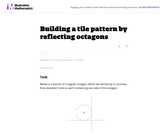Unrestricted Use
CC BY
Rating

This task applies reflections to a regular octagon to construct a pattern of four octagons enclosing a quadrilateral: the focus of the task is on using the properties of reflections to deduce that the quadrilateral is actually a square.

Subject:
Mathematics
Geometry
Material Type:
Activity/Lab
Provider:
Illustrative Mathematics
Provider Set:
Illustrative Mathematics
Author:
Illustrative Mathematics
02/09/2013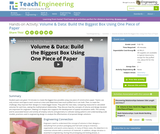Read the Fine Print
Educational Use
Rating

Student pairs are given 10 minutes to create the biggest box possible using one piece of construction paper. Teams use only scissors and tape to each construct a box and determine how much puffed rice it can hold. Then, to meet the challenge, they improve their designs to create bigger boxes. They plot the class data, comparing measured to calculated volumes for each box, seeing the mathematical relationship. They discuss how the concepts of volume and design iteration are important for engineers. Making 3-D shapes also supports the development of spatial visualization skills. This activity and its associated lesson and activity all employ volume and geometry to cultivate seeing patterns and understanding scale models, practices used in engineering design to analyze the effectiveness of proposed design solutions.

Subject:
Mathematics
Geometry
Measurement and Data
Material Type:
Activity/Lab
Provider:
TeachEngineering
Author:
Aaron Lamplugh
Malinda Zarske
Nathan Coyle
Russell Anderson
Ryan Sullivan
02/17/2021Conditional Remix & Share Permitted
CC BY-NC-SA
Rating

This book is a "flexed" version of CK-12's Basic Geometry that aligns with College Access Geometry and contains embedded literacy supports. It covers the essentials of geometry for the high school student.

Subject:
Geometry
Material Type:
Textbook
Provider:
CK-12 Foundation
Provider Set:
CK-12 FlexBook
Author:
Fauteux, Michael
Zapata, Rosamaria
05/18/2011Conditional Remix & Share Permitted
CC BY-NC-SA
Rating

Includes chapters on: Basics of Geometry, Reasoning and Proof, Parallel and Perpendicular Lines, Triangles and Congruence, Relationships with Triangles, Polygons and Quadrilaterals, Similarity, Right Triangle Trigonometry, Circles, Perimeter and Area, Surface Area and Volume, Rigid Transformations.

Subject:
Mathematics
Geometry
Material Type:
Textbook
Provider:
CK-12 Foundation
10/15/2014Conditional Remix & Share Permitted
CC BY-NC
Rating

This Geometry Concept Collection is a rigorous presentation of high school geometry. It is fully correlated with the Common Core State Standards.

Subject:
Geometry
Material Type:
Full Course
Homework/Assignment
Interactive
Lecture Notes
Lesson Plan
Textbook
Unit of Study
Provider:
CK-12 Foundation
Author:
Kaitlyn Spong
11/18/2020Conditional Remix & Share Permitted
CC BY-NC-SA
Rating

CK-12 Middle School Math Concepts for seventh grade provides a complete textbook. It presents topics including algebraic thinking, patterns, decimals, decimal operations, fractions, fraction operations, integers, integer operations, ratios, rates, proportions, percents, percent applications, equations, solving equations, inequalities, functions, graphing functions, geometry, plane geometry, solid geometry, area, perimeter, surface area, volume, statistics including mean, median, mode and range, graphing and types of graphs, and probability.

Subject:
Mathematics
Algebra
Geometry
Statistics and Probability
Material Type:
Textbook
Provider:
CK-12 Foundation
Provider Set:
CK-12 FlexBook
Author:
Jen Kershaw
11/30/2012Conditional Remix & Share Permitted
CC BY-NC-SA
Rating

CK-12 Foundation's Geometry FlexBook is a clear presentation of the essentials of geometry for the high school student. Topics include: Proof, Congruent Triangles, Quadrilaterals, Similarity, Perimeter & Area, Volume, and Transformations.

Subject:
Geometry
Material Type:
Textbook
Provider:
CK-12 Foundation
Provider Set:
CK-12 FlexBook
10/06/2009Conditional Remix & Share Permitted
CC BY-NC-SA
Rating

Find a career related to geometry and create a geometry problem that is based on that career.

Subject:
Geometry
Material Type:
Lesson Plan
Author:
Tyler Schultz
07/29/2019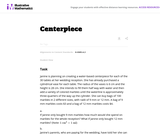Unrestricted Use
CC BY
Rating

The purpose of this task is to use geometric and algebraic reasoning to model a real-life scenario. In particular, students are in several places (implicitly or explicitly) to reason as to when making approximations is reasonable and when to round, when to use equalities vs. inequalities, and the choice of units to work with (e.g., mm vs. cm).

Subject:
Mathematics
Geometry
Material Type:
Activity/Lab
Provider:
Illustrative Mathematics
Provider Set:
Illustrative Mathematics
Author:
Illustrative Mathematics
05/01/2012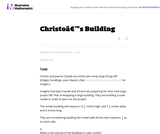Unrestricted Use
CC BY
Rating

This task is primarily about volume and surface area, although it also gives students an early look at converting between measurements in scale models and the real objects they correspond to.

Subject:
Mathematics
Geometry
Material Type:
Activity/Lab
Provider:
Illustrative Mathematics
Provider Set:
Illustrative Mathematics
Author:
Illustrative Mathematics
05/01/2012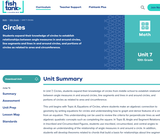Conditional Remix & Share Permitted
CC BY-NC-SA
Rating

Students expand their knowledge of circles to establish relationships between angle measures in and around circles, line segments and lines in and around circles, and portions of circles as related to area and circumference.

Subject:
Mathematics
Geometry
Material Type:
Unit of Study
Provider:
Fishtank Learning
Provider Set:
Mathematics
11/19/2021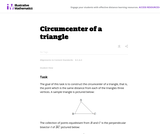Unrestricted Use
CC BY
Rating

This task shows that the three perpendicular bisectors of the sides of a triangle all meet in a point, using the characterization of the perpendicular bisector of a line segment as the set of points equidistant from the two ends of the segment. The point so constructed is called the circumcenter of the triangle.

Subject:
Mathematics
Geometry
Material Type:
Activity/Lab
Provider:
Illustrative Mathematics
Provider Set:
Illustrative Mathematics
Author:
Illustrative Mathematics
03/04/2013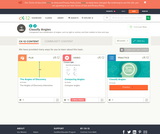Conditional Remix & Share Permitted
CC BY-NC-SA
Rating

This short video and interactive assessment activity is designed to give fourth graders an overview of angles.

Subject:
Mathematics
Geometry
Material Type:
Assessment
Interactive
Lecture
Provider:
CK-12 Foundation
Provider Set:
CK-12 Elementary Math
11/18/2020Conditional Remix & Share Permitted
CC BY-NC-SA
Rating

This short video and interactive assessment activity is designed to teach third graders about classifying all types of triangles by lengths of sides.

Subject:
Mathematics
Geometry
Material Type:
Assessment
Interactive
Lecture
Provider:
CK-12 Foundation
Provider Set:
CK-12 Elementary Math
11/18/2020Conditional Remix & Share Permitted
CC BY-NC-SA
Rating

This short video and interactive assessment activity is designed to teach fourth graders about classifying all types of triangles by lengths of sides.

Subject:
Mathematics
Geometry
Material Type:
Assessment
Interactive
Lecture
Provider:
CK-12 Foundation
Provider Set:
CK-12 Elementary Math
11/18/2020Conditional Remix & Share Permitted
CC BY-NC-SA
Rating

This short video and interactive assessment activity is designed to teach third graders an overview of triangle classifications.

Subject:
Mathematics
Geometry
Material Type:
Assessment
Interactive
Lecture
Provider:
CK-12 Foundation
Provider Set:
CK-12 Elementary Math
11/18/2020Conditional Remix & Share Permitted
CC BY-NC-SA
Rating

This short video and interactive assessment activity is designed to teach third graders about classifying triangles based on equal sides.

Subject:
Mathematics
Geometry
Material Type:
Assessment
Interactive
Lecture
Provider:
CK-12 Foundation
Provider Set:
CK-12 Elementary Math
11/18/2020Conditional Remix & Share Permitted
CC BY-NC-SA
Rating

This short video and interactive assessment activity is designed to teach fourth graders about classifying triangles based on equal sides.

Subject:
Mathematics
Geometry
Material Type:
Assessment
Interactive
Lecture
Provider:
CK-12 Foundation
Provider Set:
CK-12 Elementary Math
11/18/2020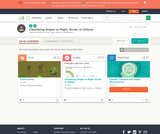Conditional Remix & Share Permitted
CC BY-NC-SA
Rating

This short video and interactive assessment activity is designed to give fourth graders an overview of triangle classifications.

Subject:
Mathematics
Geometry
Material Type:
Assessment
Interactive
Lecture
Provider:
CK-12 Foundation
Provider Set:
CK-12 Elementary Math
11/18/2020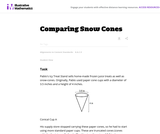Unrestricted Use
CC BY
Rating

Students must calculate the volume of a cone in this real world task.

Subject:
Mathematics
Geometry
Material Type:
Activity/Lab
Provider:
Illustrative Mathematics
Provider Set:
Illustrative Mathematics
Author:
Illustrative Mathematics
05/01/2012Conditional Remix & Share Permitted
CC BY-NC-SA
Rating

This short video and interactive assessment activity is designed to give fourth graders an overview of forming figures with cutouts.

Subject:
Mathematics
Geometry
Material Type:
Assessment
Interactive
Lecture
Provider:
CK-12 Foundation
Provider Set:
CK-12 Elementary Math
11/18/2020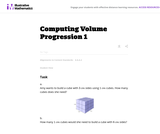Unrestricted Use
CC BY
Rating

The purpose of this series of tasks is to build in a natural way from accessible, concrete problems involving volume to a more abstract understanding of volume. The purpose of this first task is to see the relationship between the side-lengths of a cube and its volume.

Subject:
Mathematics
Geometry
Material Type:
Activity/Lab
Provider:
Illustrative Mathematics
Provider Set:
Illustrative Mathematics
Author:
Illustrative Mathematics
05/01/2012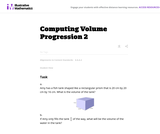Unrestricted Use
CC BY
Rating

The purpose of this series of tasks is to build in a natural way from accessible, concrete problems involving volume to a more abstract understanding of volume. In this iteration, we do away with the lines that delineate individual unit cubes (which makes it more abstract) and generalize from cubes to rectangular prisms.

Subject:
Mathematics
Geometry
Material Type:
Activity/Lab
Provider:
Illustrative Mathematics
Provider Set:
Illustrative Mathematics
Author:
Illustrative Mathematics
05/01/2012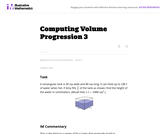Unrestricted Use
CC BY
Rating

The purpose of this series of tasks is to build in a natural way from accessible, concrete problems involving volume to a more abstract understanding of volume. Here, we are given the volume and are asked to find the height.

Subject:
Mathematics
Geometry
Material Type:
Activity/Lab
Provider:
Illustrative Mathematics
Provider Set:
Illustrative Mathematics
Author:
Illustrative Mathematics
05/01/2012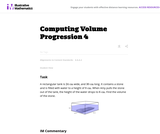Unrestricted Use
CC BY
Rating

The purpose of this series of tasks is to build in a natural way from accessible, concrete problems involving volume to a more abstract understanding of volume. This problem is based on ArchimedesŐ Principle that the volume of an immersed object is equivalent to the volume of the displaced water.

Subject:
Mathematics
Geometry
Material Type:
Activity/Lab
Provider:
Illustrative Mathematics
Provider Set:
Illustrative Mathematics
Author:
Illustrative Mathematics
05/01/2012Read the Fine Print
Educational Use
Rating

In this video segment you&rsquo;ll discover how using concentric circles can help you determine how much paint is needed to cover the deck of a carousel.

Subject:
Mathematics
Geometry
Material Type:
Lesson
Provider:
PBS LearningMedia
Author:
New York Network
WNET
05/13/2010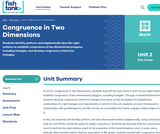Conditional Remix & Share Permitted
CC BY-NC-SA
Rating

Students identify, perform, and algebraically describe rigid motions to establish congruence of two dimensional polygons, including triangles, and develop congruence criteria for triangles.

Subject:
Mathematics
Geometry
Material Type:
Unit of Study
Provider:
Fishtank Learning
Provider Set:
Mathematics
11/19/2021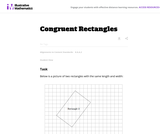Unrestricted Use
CC BY
Rating

This task is designed to give students insight into the effects of translations, rotations, and reflections on geometric figures in the context of showing that two figures are congruent.

Subject:
Mathematics
Geometry
Material Type:
Activity/Lab
Provider:
Illustrative Mathematics
Provider Set:
Illustrative Mathematics
Author:
Illustrative Mathematics